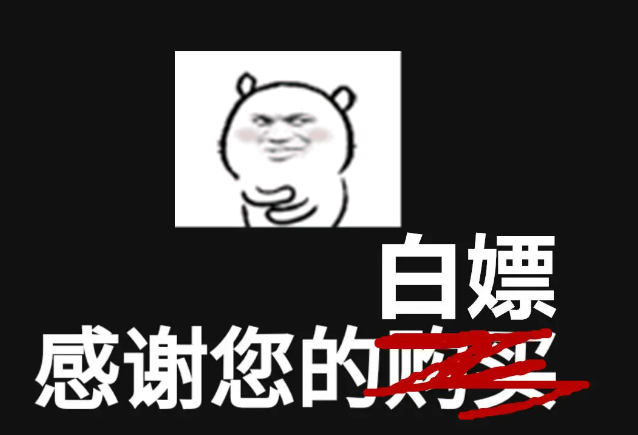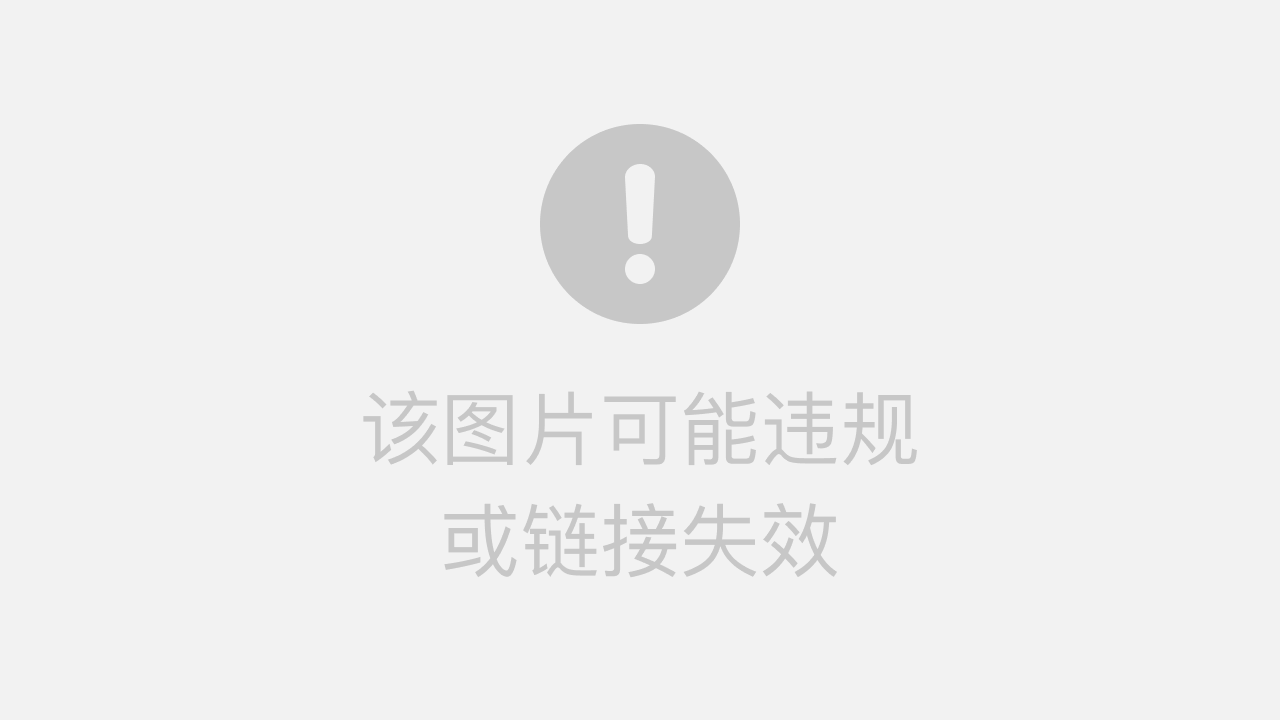# 前言Python的小技巧很多，入门容易精通难！在进阶的路上，有没有什么好的技巧和好的方法，就

## 所有文章完整的素材+源码都在👇👇# 正文

1）01 for循环中的else条件

``````numbers = [2, 4, 6, 8, 1]
for number in numbers:
if number % 2 == 1:
print(number)
break
else:
print('没有数字是奇数')``````

2）02 从列表中获取元素,定义多个变量

``````my_list = [1,2,3,4,5]
a, b, c, d, e = my_list
print(a)
print(b)
print(c)
print(d)
print(e)``````3）3 使用heapq模块，获取列表中n个最大或最小的元素

``````import heapq

scores = [51, 33, 64, 87, 91, 75, 15, 49, 33, 82]
print(heapq.nsmallest(5, scores))
print(heapq.nlargest(2, scores))``````4）4、将列表中的所有元素作为参数传递给函数

``````my_list = [1,2,3,4]

def sum_of_elements(*arg):
total = 0
for i in arg:
total += i

print(sum_of_elements(*my_list))``````5）​5、列表推导式

``````numbers = [1,2,3,4,5]
s_numbers = [num * num for num in numbers]
print(s_numbers)``````6）​ 6、重复字符串：从列表中获取元素,定义多个变量

``````my_list = [1,2,3,4,5]
a, b, c, d, e = my_list
print(a)
print(b)
print(c)
print(d)
print(e)
print('-' * 100)
# 4、将列表中的所有元素作为参数传递给函数
my_list = [1,2,3,4]
def sum_of_elements(*arg):
total = 0
for i in arg:
total += i
print(sum_of_elements(*my_list))``````7）7 字符串转列表或者字典

``````list_str = '[1,2,3,4,5]'
dict_str = '{"A": "啊", "B": "不"}'
int_str = '1'
print(eval(list_str))
print(type(eval(list_str)))
print(eval(dict_str))
print(type(eval(dict_str)))
print(eval(int_str))
print(type(eval(int_str)))

# json数据转字典

import json说明：

# 总结

``（附带小抄：1、1 2 3 4 5 、[15, 33, 33, 49, 51] [91, 87] 、10 、[1, 4, 9, 16, 25] 、 1 2 3 4 5 ---------------------------------------------------------------------------------------------------- 10 、[1, 2, 3, 4, 5] <class 'list'> {'A': '啊', 'B': '不'} <class 'dict'> 1 <class 'int'> <class 'dict'> ）``

# 🎁文章汇总——

Python文章合集 | (入门到实战、游戏、Turtle、案例等)

（文章汇总还有更多你案例等你来学习啦~源码找我即可免费！）